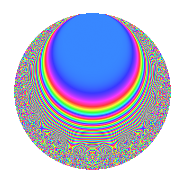# Properties

 Label 354.4.aLevel 354 Weight 4 Character orbit a Rep. character $$\chi_{354}(1,\cdot)$$ Character field $$\Q$$ Dimension 28 Newform subspaces 9 Sturm bound 240 Trace bound 5

# Related objects

## Defining parameters

 Level: $$N$$ = $$354 = 2 \cdot 3 \cdot 59$$ Weight: $$k$$ = $$4$$ Character orbit: $$[\chi]$$ = 354.a (trivial) Character field: $$\Q$$ Newform subspaces: $$9$$ Sturm bound: $$240$$ Trace bound: $$5$$ Distinguishing $$T_p$$: $$5$$

## Dimensions

The following table gives the dimensions of various subspaces of $$M_{4}(\Gamma_0(354))$$.

Total New Old
Modular forms 184 28 156
Cusp forms 176 28 148
Eisenstein series 8 0 8

The following table gives the dimensions of the cuspidal new subspaces with specified eigenvalues for the Atkin-Lehner operators and the Fricke involution.

$$2$$$$3$$$$59$$FrickeDim.
$$+$$$$+$$$$+$$$$+$$$$3$$
$$+$$$$+$$$$-$$$$-$$$$3$$
$$+$$$$-$$$$+$$$$-$$$$2$$
$$+$$$$-$$$$-$$$$+$$$$5$$
$$-$$$$+$$$$+$$$$-$$$$3$$
$$-$$$$+$$$$-$$$$+$$$$4$$
$$-$$$$-$$$$+$$$$+$$$$6$$
$$-$$$$-$$$$-$$$$-$$$$2$$
Plus space$$+$$$$18$$
Minus space$$-$$$$10$$

## Trace form

 $$28q + 4q^{2} + 6q^{3} + 112q^{4} - 12q^{5} + 44q^{7} + 16q^{8} + 252q^{9} + O(q^{10})$$ $$28q + 4q^{2} + 6q^{3} + 112q^{4} - 12q^{5} + 44q^{7} + 16q^{8} + 252q^{9} + 40q^{10} + 112q^{11} + 24q^{12} + 8q^{13} - 48q^{15} + 448q^{16} + 324q^{17} + 36q^{18} - 96q^{19} - 48q^{20} - 96q^{21} - 232q^{22} - 208q^{23} + 1268q^{25} - 152q^{26} + 54q^{27} + 176q^{28} + 12q^{29} + 48q^{30} + 68q^{31} + 64q^{32} + 192q^{33} + 152q^{34} + 104q^{35} + 1008q^{36} - 544q^{37} + 208q^{38} + 252q^{39} + 160q^{40} - 356q^{41} + 92q^{43} + 448q^{44} - 108q^{45} + 64q^{46} + 584q^{47} + 96q^{48} + 1560q^{49} - 580q^{50} + 36q^{51} + 32q^{52} - 412q^{53} + 1584q^{55} + 888q^{57} + 136q^{58} - 192q^{60} + 3608q^{61} + 32q^{62} + 396q^{63} + 1792q^{64} + 1848q^{65} - 768q^{66} - 332q^{67} + 1296q^{68} + 1248q^{69} + 144q^{70} + 1864q^{71} + 144q^{72} + 1664q^{73} + 1688q^{74} + 426q^{75} - 384q^{76} + 1496q^{77} - 312q^{78} - 1748q^{79} - 192q^{80} + 2268q^{81} + 648q^{82} - 1288q^{83} - 384q^{84} + 1752q^{85} + 48q^{86} + 1152q^{87} - 928q^{88} - 5468q^{89} + 360q^{90} + 1392q^{91} - 832q^{92} + 36q^{93} - 2672q^{94} - 4768q^{95} - 2336q^{97} - 860q^{98} + 1008q^{99} + O(q^{100})$$

## Decomposition of $$S_{4}^{\mathrm{new}}(\Gamma_0(354))$$ into newform subspaces

Label Dim. $$A$$ Field CM Traces A-L signs $q$-expansion
$$a_2$$ $$a_3$$ $$a_5$$ $$a_7$$ 2 3 59
354.4.a.a $$2$$ $$20.887$$ $$\Q(\sqrt{21})$$ None $$-4$$ $$6$$ $$-12$$ $$-23$$ $$+$$ $$-$$ $$+$$ $$q-2q^{2}+3q^{3}+4q^{4}+(-5-2\beta )q^{5}+\cdots$$
354.4.a.b $$2$$ $$20.887$$ $$\Q(\sqrt{51})$$ None $$-4$$ $$6$$ $$26$$ $$0$$ $$+$$ $$-$$ $$-$$ $$q-2q^{2}+3q^{3}+4q^{4}+(13+\beta )q^{5}+\cdots$$
354.4.a.c $$2$$ $$20.887$$ $$\Q(\sqrt{13})$$ None $$4$$ $$6$$ $$-20$$ $$-23$$ $$-$$ $$-$$ $$-$$ $$q+2q^{2}+3q^{3}+4q^{4}+(-9-2\beta )q^{5}+\cdots$$
354.4.a.d $$3$$ $$20.887$$ 3.3.30645.1 None $$-6$$ $$-9$$ $$-6$$ $$6$$ $$+$$ $$+$$ $$-$$ $$q-2q^{2}-3q^{3}+4q^{4}+(-2-\beta _{1}-2\beta _{2})q^{5}+\cdots$$
354.4.a.e $$3$$ $$20.887$$ 3.3.45581.1 None $$-6$$ $$-9$$ $$4$$ $$13$$ $$+$$ $$+$$ $$+$$ $$q-2q^{2}-3q^{3}+4q^{4}+(2-\beta _{1}+\beta _{2})q^{5}+\cdots$$
354.4.a.f $$3$$ $$20.887$$ 3.3.18989.1 None $$-6$$ $$9$$ $$-28$$ $$26$$ $$+$$ $$-$$ $$-$$ $$q-2q^{2}+3q^{3}+4q^{4}+(-9+\beta _{1}+\beta _{2})q^{5}+\cdots$$
354.4.a.g $$3$$ $$20.887$$ 3.3.47277.1 None $$6$$ $$-9$$ $$-18$$ $$6$$ $$-$$ $$+$$ $$+$$ $$q+2q^{2}-3q^{3}+4q^{4}+(-6+\beta _{1}+\beta _{2})q^{5}+\cdots$$
354.4.a.h $$4$$ $$20.887$$ $$\mathbb{Q}[x]/(x^{4} - \cdots)$$ None $$8$$ $$-12$$ $$22$$ $$13$$ $$-$$ $$+$$ $$-$$ $$q+2q^{2}-3q^{3}+4q^{4}+(5+\beta _{1}-\beta _{3})q^{5}+\cdots$$
354.4.a.i $$6$$ $$20.887$$ $$\mathbb{Q}[x]/(x^{6} - \cdots)$$ None $$12$$ $$18$$ $$20$$ $$26$$ $$-$$ $$-$$ $$+$$ $$q+2q^{2}+3q^{3}+4q^{4}+(3+\beta _{1})q^{5}+\cdots$$

## Decomposition of $$S_{4}^{\mathrm{old}}(\Gamma_0(354))$$ into lower level spaces

$$S_{4}^{\mathrm{old}}(\Gamma_0(354)) \cong$$ $$S_{4}^{\mathrm{new}}(\Gamma_0(6))$$$$^{\oplus 2}$$$$\oplus$$$$S_{4}^{\mathrm{new}}(\Gamma_0(59))$$$$^{\oplus 4}$$$$\oplus$$$$S_{4}^{\mathrm{new}}(\Gamma_0(118))$$$$^{\oplus 2}$$$$\oplus$$$$S_{4}^{\mathrm{new}}(\Gamma_0(177))$$$$^{\oplus 2}$$